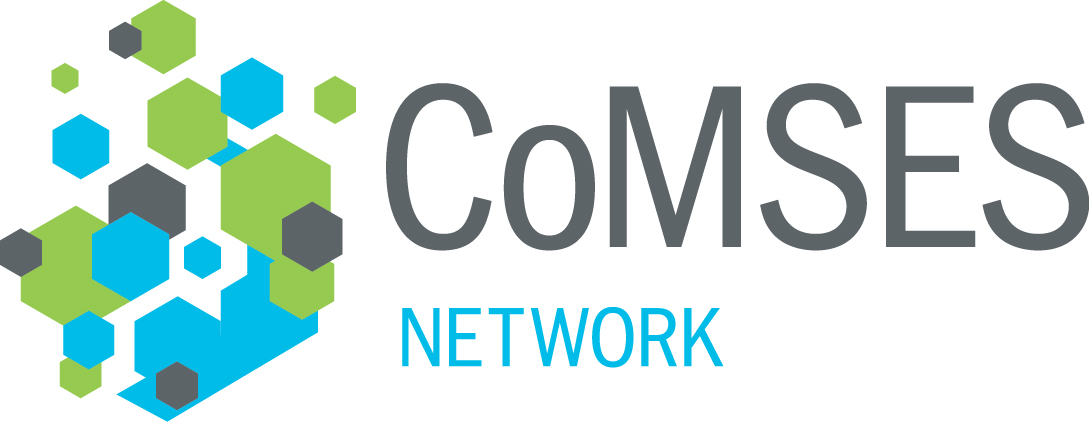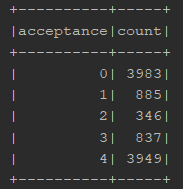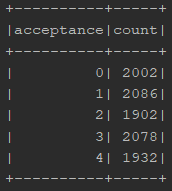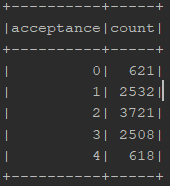# Any work on Sociotropy-Autonomy Scale?

Is anyone aware of any prior work creating a computational model of Bieling, Beck, Brown (2000) ?

Here is something I mocked with weighted averages:

``````   def adjustParams(sociotropy: Double, autonomy: Double) =
DecisionParams(
(param.selfParams._1, param.selfParams._2, param.selfParams._3 * autonomy),
param.groupPreferences,
param.groupWeights.map(weights => weights._1 -> weights._2 * sociotropy / groupSize)
)
def calculate: Boolean = (selfWsum + groupWsum) / (groupWeightsSum + selfWeight) > 0.5
}
``````

Tested out with my Abilene toy model, with the assumption that distribution of ‘yes votes’ would be a beta distribution with α = β = sociotropy:autonomy e.g. higher sociotropy would yield more likely decision consensus and vice versa.

Acceptance represents count of yes votes for a given group and count is the count of that number of votes out of 10000 simulated. (Left to right: α = β = 9999, α = β = 1, α = β = 1/9999)Since the initial assumption checks out I am assuming the two high level components of the scale can be modeled with this approach.